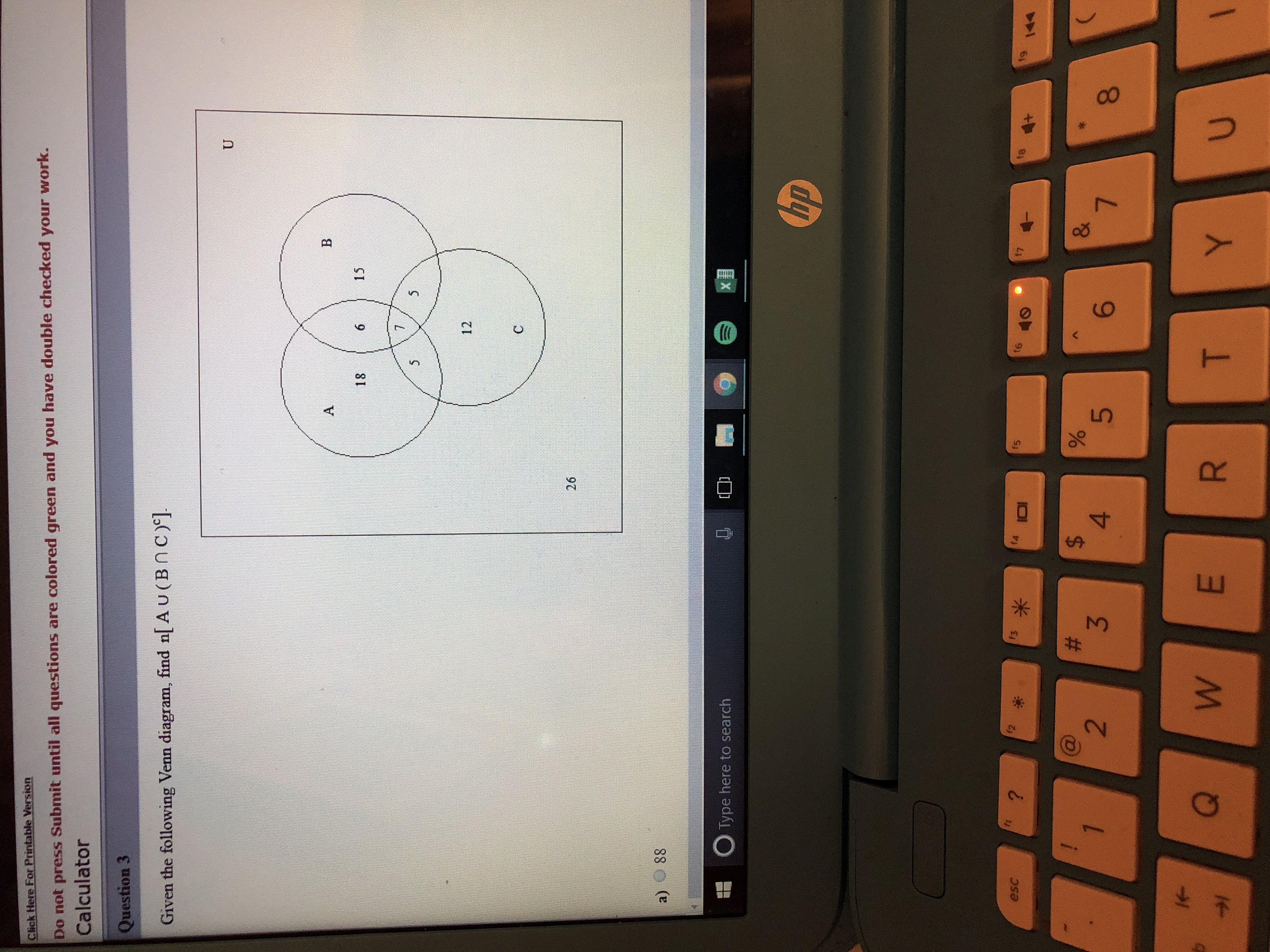# lick Here For Printable VersionDo not press Submit until all questions are colored green and you have double checked your work.CalculatorQuestion 3Given the following Venn diagram, find n[ A u ( B n C )18 6151226a) 88Type here to search(upescfe23468

Question
1 viewshelp_outlineImage Transcriptioncloselick Here For Printable Version Do not press Submit until all questions are colored green and you have double checked your work. Calculator Question 3 Given the following Venn diagram, find n[ A u ( B n C ) 18 615 12 26 a) 88 Type here to search (up esc fe 2 3 4 6 8 fullscreen
check_circle

Step 1

Calculation:

Shade  (B Ո C) in the given venn diagram.

In general intersection set of two set will contain only the common elements.

Thus, shade the regions which belongs to both the set A and B as shown below.

Step 2

Now obtain the region of (B Ո C)c

In general, the complement of a set is the region that is not in the set (B Ո C).

That is, U- (B Ո C).

Thus, the region of(B Ո C)c in the given venn diagram is shown below.

Step 3

Compute the region of [A Ս (b Ո C)^c].

In general, the union set of two sets contaoins all the elements of both...

### Want to see the full answer?

See Solution

#### Want to see this answer and more?

Solutions are written by subject experts who are available 24/7. Questions are typically answered within 1 hour.*

See Solution
*Response times may vary by subject and question.
Tagged in

### Math